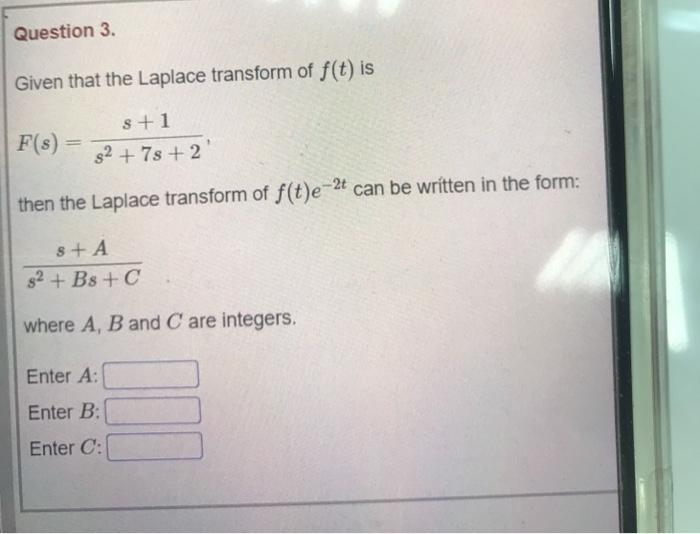### Create an Account

Already have account?

### Forgot Your Password ?

Home / Questions / Question 3. Given that the Laplace transform of f(t) is F(s) s +1 82 +7s+2' then the Lapla...

# Question 3. Given that the Laplace transform of f(t) is F(s) s +1 82 +7s+2' then the Laplace transform of f(t)e-2t can be written in the form: sta 82 + B8 + where A, B and C are integers. Enter A: Ent

Question 3. Given that the Laplace transform of f(t) is F(s) s +1 82 +7s+2' then the Laplace transform of f(t)e-2t can be written in the form: sta 82 + B8 + where A, B and C are integers. Enter A: Enter B: Enter C:Apr 15 2021 View more View Less

#### Answer (Solved)Subscribe To Get Solution